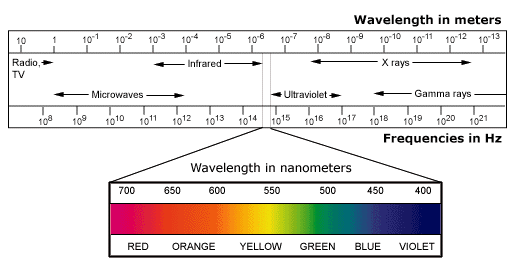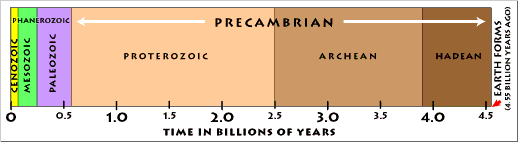Geologic context:
radioactive decay and geologic age, geologic time, metric system

# Big Numbers and Scientific Notation

by Jennifer M. Wenner, Geology Department, University of Wisconsin-Oshkosh
Jump down to: Teaching strategies | Materials & Exercises | Student Resources

## What is scientific notation?

The concept of very large or very small numbers is something that is difficult for many students to comprehend. In general, students have difficulty with two things when dealing with numbers that have more zeros (either before OR after the decimal point) than they are used to. They often do not understand:

1. that "big" and "small" are relative terms,
2. the concept of "order of magnitude"

Scientific notation is a way to assess the order of magnitude and to visually decrease the zeros that the student sees. It also may help students to compare very large (or very small numbers) But students still have little intuition about scientific notation. Teaching them to recognize that scientific notation is a short hand way to better understand big and small numbers can be useful to them in all aspects of their academic career.

In science, often we work with very large or very small numbers. For example, in geology,

the age of the Earth = 4,600,000,000 years old,
or, in chemistry,
one a.m.u. = 0.00000000000000000000000000166 kilograms.
It seems like a lot of work to keep track of all those zeros. Fortunately, we can easily keep track of zeros and compare the size of numbers with scientific notation.

Scientific notation allows us to reduce the number of zeros that we see while still keeping track of them for us. For example the age of the Earth (see above) can be written as 4.6 X 109 years. This means that this number has 9 places after the decimal place - filled with zeros unless a number comes after the decimal when writing scientific notation. So 4.6 X 109 years represents 4600000000 years.

Very small numbers use the same type of notation only the exponent on the 10 is usually a negative number. For example, 0.00000000000000000000000000166 kg (the weight of one atomic mass unit (a.m.u.)) would be written 1.66 x 10-27 using scientific notation. A negative number after the 10 means that we count places before the decimal point in the scientific notation. You can count how many numbers are between the decimal point in the first number and the second number and it should equal 27.

The nice thing about scientific notation is that it also tells us something about significant figures.

## Teaching Strategies: Ideas from Math Education

### Put quantitative concepts in context

There are a number of geologic contexts in which big (and small) numbers and scientific notation come up. A few of them include:

### Use multiple representations

Because everyone has different ways of learning, mathematicians have defined a number of ways that quantitative concepts can be represented to individuals. Here are some ways that big numbers and scientific notation can be represented.

• Numerical representation
• 4.5 billion years looks like this:
4500000000 years or 4,500,000,000 years
That's a lot of zeros!
We can also write that number in scientific notation:
4.5 X 109 yrsor (on a calculator) 4.5E9 yrs
(1 billion in scientific notation means
10 x 10 x 10 x 10 x 10 x 10 x 10 x 10 x 10!)
What does that number actually mean? Can you actually imagine 4.5 billion of anything?
• Graphical representation
• Although many topics in geoscience use scientific notation, graphical representations are often plotted on logarithmic scales as in the electromagnetic spectrum below.Log scale of electromagnetic spectrum. Details

There are many reasons for this - most importantly, it takes up less space - but a log scale does not give a sense of true scale:

Geologic timeis a great context in which to talk about big numbers and to give a sense of scale. We have the geologic time scale to give students a sense of what parts of "time" we know the most about. And how big "time" really is.

Here is a geologic time scale that gives a sense of scaleGeologic time scale. Details

It is interesting to note that the time periods that we know the most about (the Cenozoic, Mesozoic and Paleozoic) only make up a small fraction of the whole of geologic time!
• Symbolic representation
• There are a number of analogies for thinking about big numbers. Students (particularly in entry-level classes) often relate well to analogies with money:
Money analogies:
• A local radio station is tring to collect a million pennies for a worthy cause. How many dollars is this?
• You have just won a contest. The prize is that you will get \$1 bills from a bank teller at the rate of 1 per second for as long as you can stand there taking them (no bathroom breaks, no napping, etc.). How long will you have to stand there to become a millionaire?
• A very rich man decides that he will help to pay off the national debt by paying \$1 into the coffers of the US at the rate of one per second. How long will it take him to pay off the debt (provided no more debt accumulates). (You can check the debt (to the penny) at the Treasury Dept. - over 7.6 trillion dollars in Feb 2005)?

### Use technology appropriately

Students have a number of tools that can be used to help them become more familiar with both scientific notation and very large and very small numbers. Calculators can be set up to display scientific notation. Spreadsheet programs can tabulate data, expressing it in a number of forms.
• Calculators:
• Scientific notation provides an excellent way for students to become familiar with their (probably new) calculators. Many students do not know what scientific notation is and have little intuition about it. Even fewer really know how to use their calculators to express it. Often I see students just ignoring "the little number in the upper right" and so their answer is off by orders of magnitude. Have students figure out how to make their calculators express scientific notation. A quick exercise to do in class is have them multiply some numbers in scientific notation on their calculator and then write the answer in numerical notation.
• Computers:
• Many spreadsheets can be formatted to display numbers numerically and in scientific notation. Showing students a table of these data expressed both ways may help them to get a sense of what is happening "in their calculators" when numbers are displayed in scientific notation. These numbers can also be plotted so that students can get a sense of scale.

### Work in groups to do multiple day, in-depth problems

Mathematicians also indicate that students learn quantitative concepts better when they work in groups and revisit a concept on more than one day. Therefore, when discussing quantitative concepts in entry-level geoscience courses, have students discuss or practice the concepts together. Also, make sure that you either include problems that may be extended over more than one class period or revisit the concept on numerous occasions.

Very big and very small numbers come up over and over in introductory geoscience: Geologic time, radiometric dating, unit conversions (especially metric), etc. When each new topic is introduced, make sure to point out that this is not new material, it's just a matter of remembering.

## Teaching Materials and Exercises

• Jerry Johnson of University of Nevada at Reno has an excellent example of Understanding Big Numbers that applies to population growth and government spending. This file includes student exercises and instructor notes.
• In the Quantitative Skills site, Barb Tewksbury discusses Back of the Envelope Calculations and has a number of exercises that give students practice in quantitative skills and in estimating numbers.
• A View from the Back of the Envelope has a number of links to interesting ways to explain (and estimate) big numbers and orders of magnitude.

## Student resources

MathWorld has a more mathematical explanation of scientific notation with a link to significant digits .